Скачать презентацию Chapter 10 Managing Costs Management by

ffdcea689ff25928868bb573944c9503.ppt

• Количество слайдов: 69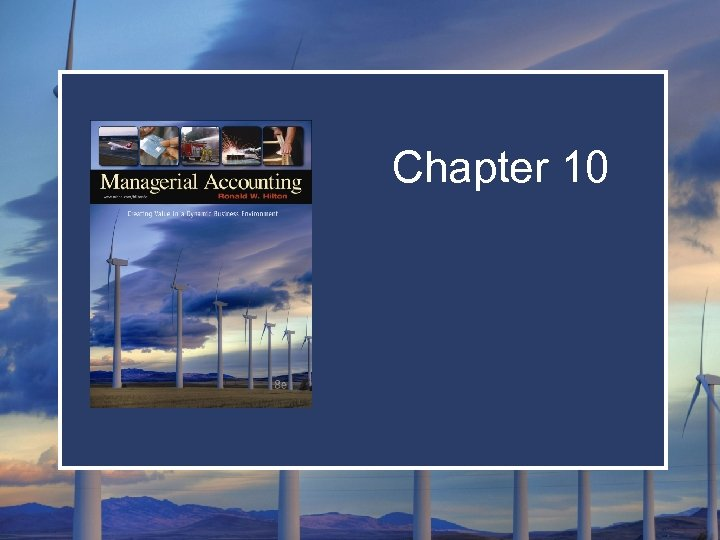Chapter 10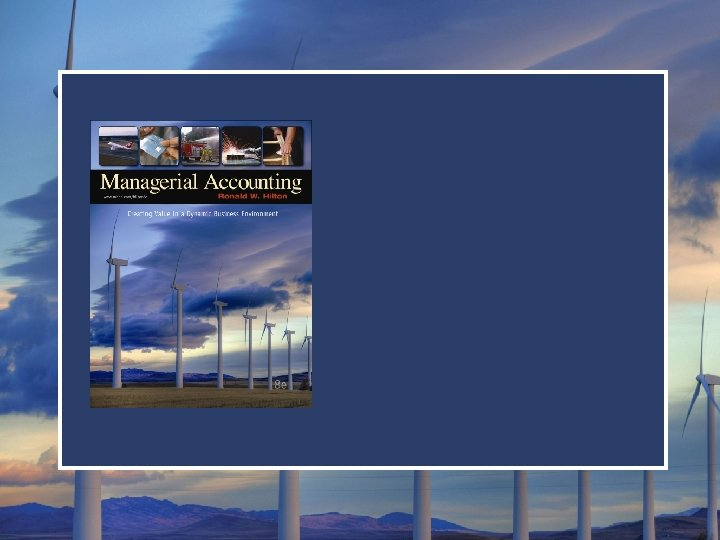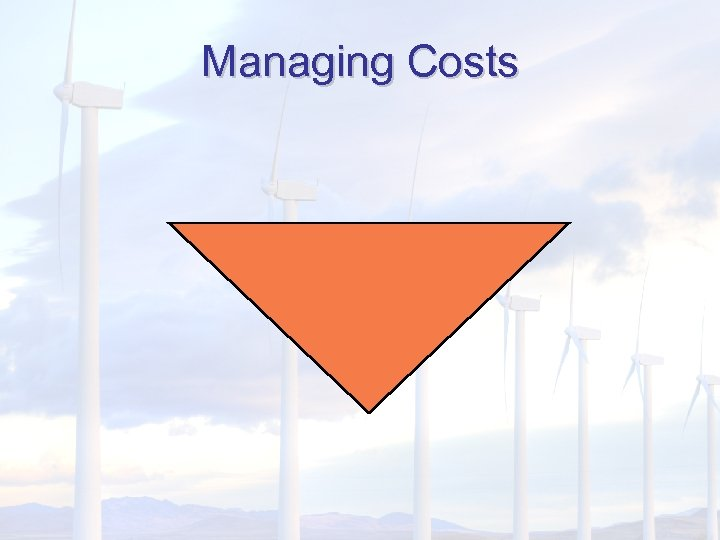Managing Costs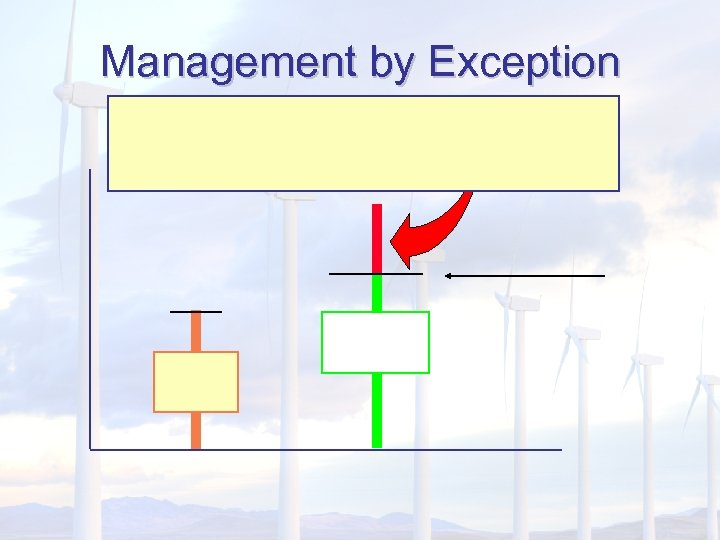Management by Exception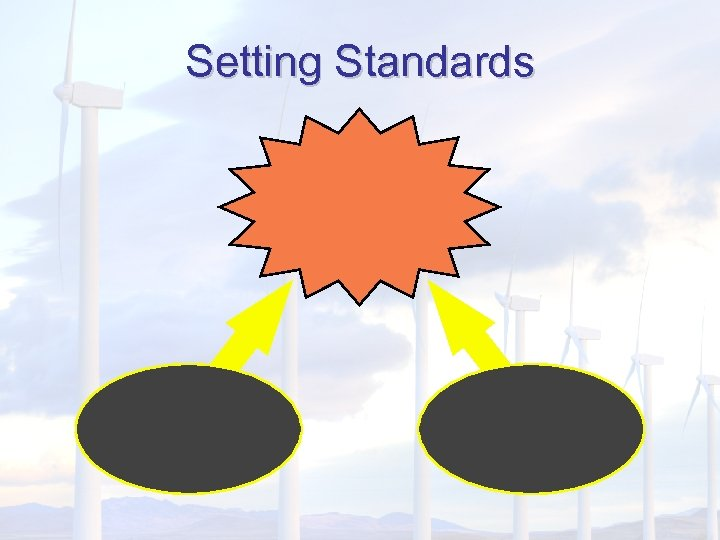Setting StandardsParticipation in Setting Standards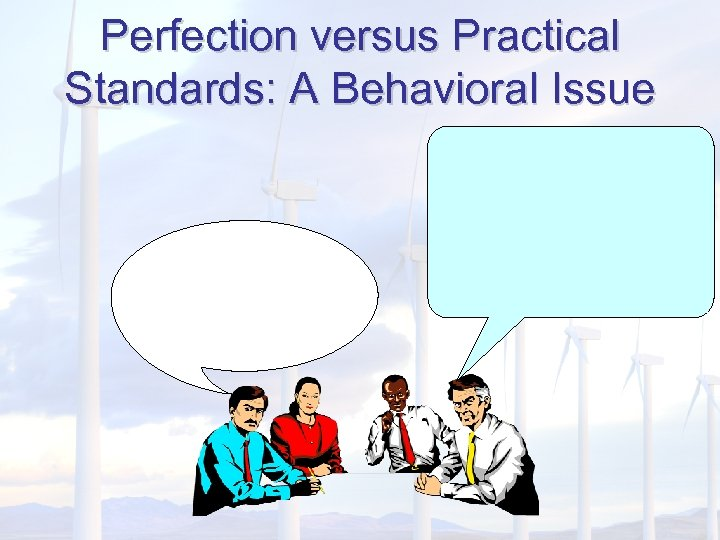Perfection versus Practical Standards: A Behavioral Issue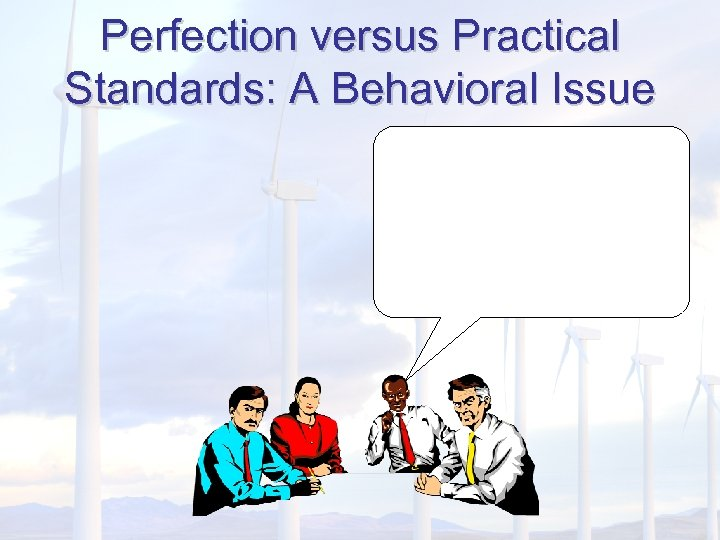Perfection versus Practical Standards: A Behavioral Issue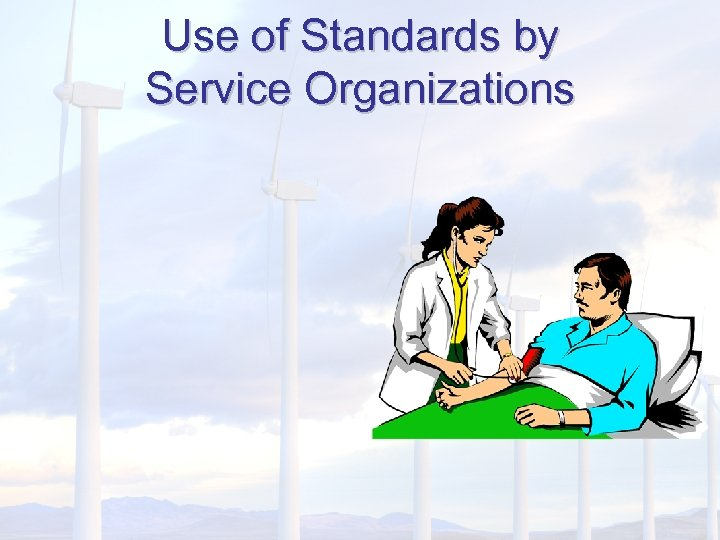Use of Standards by Service Organizations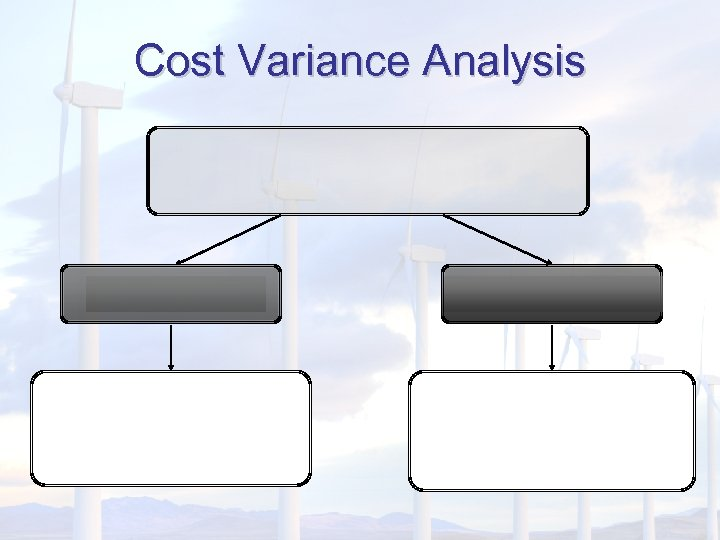Cost Variance Analysis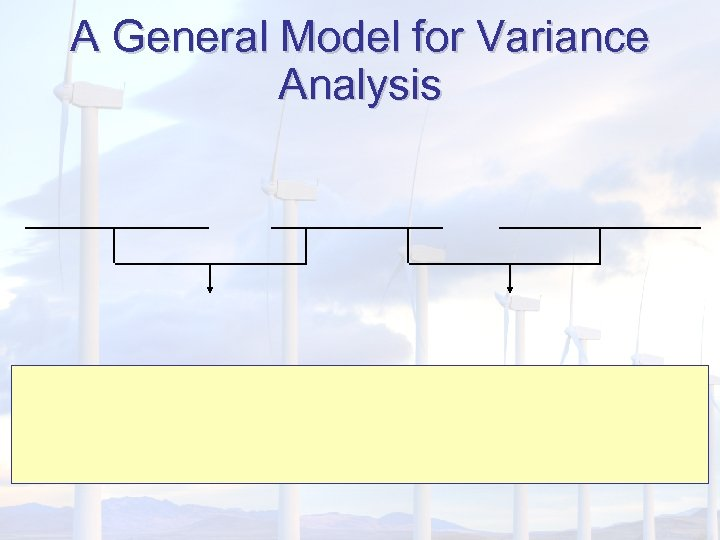A General Model for Variance Analysis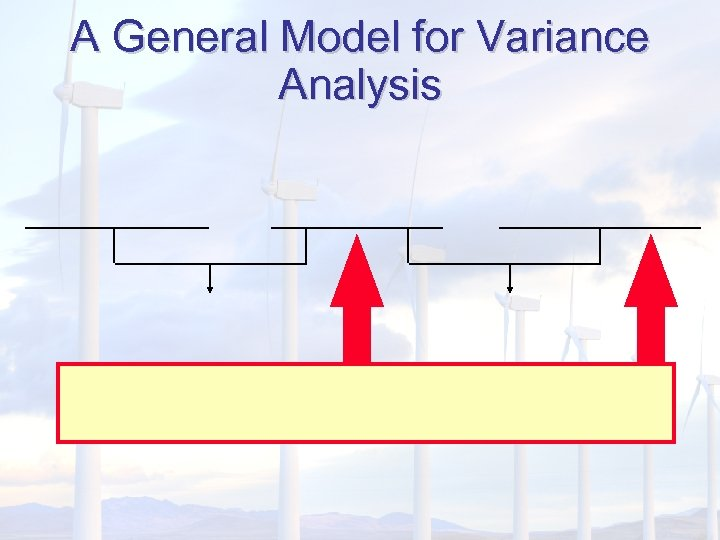A General Model for Variance Analysis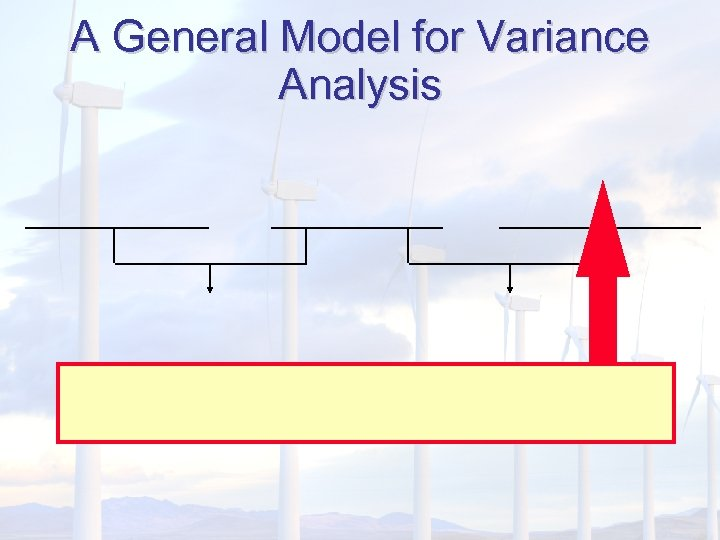A General Model for Variance Analysis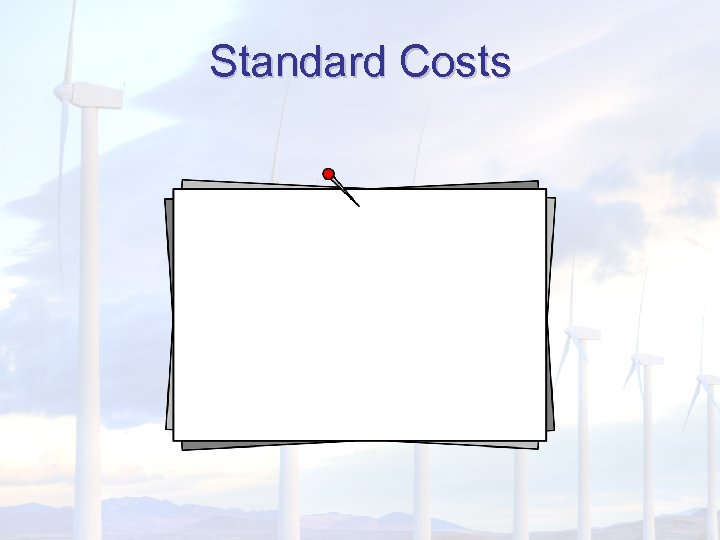Standard Costs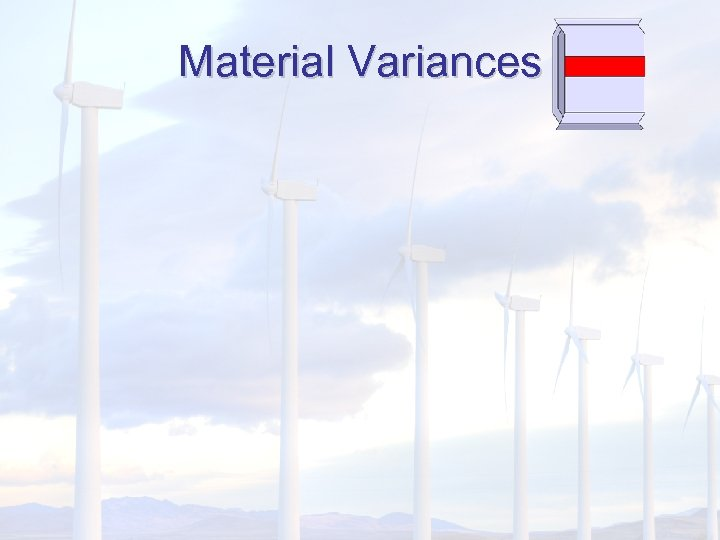Material Variances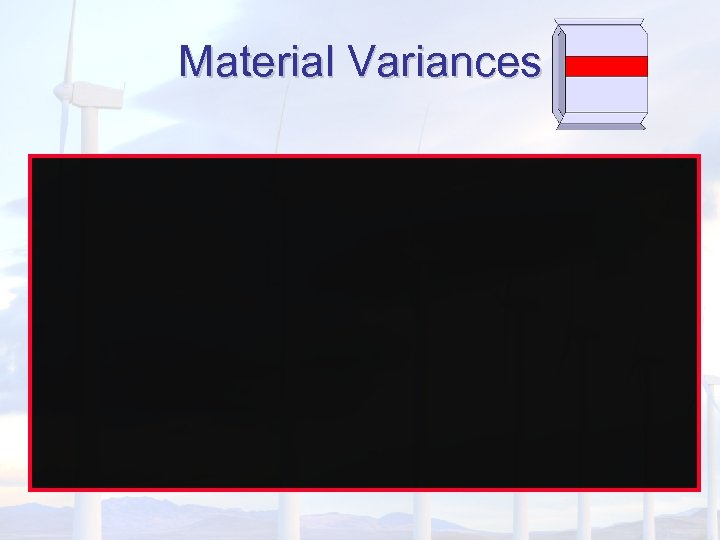Material Variances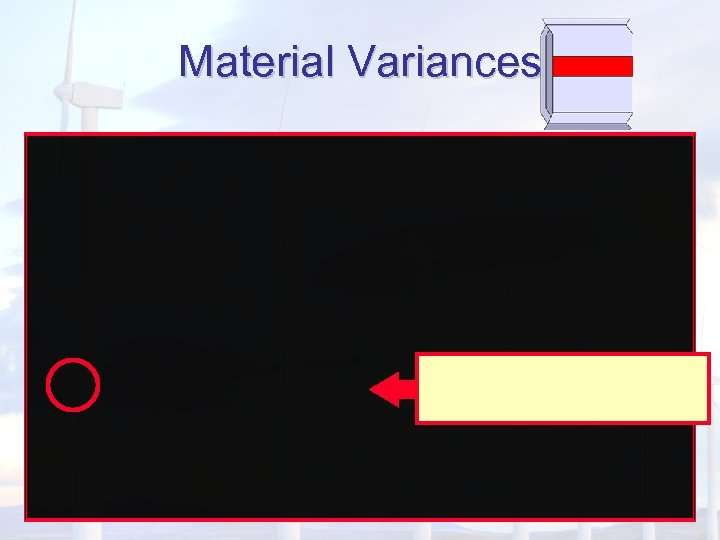Material Variances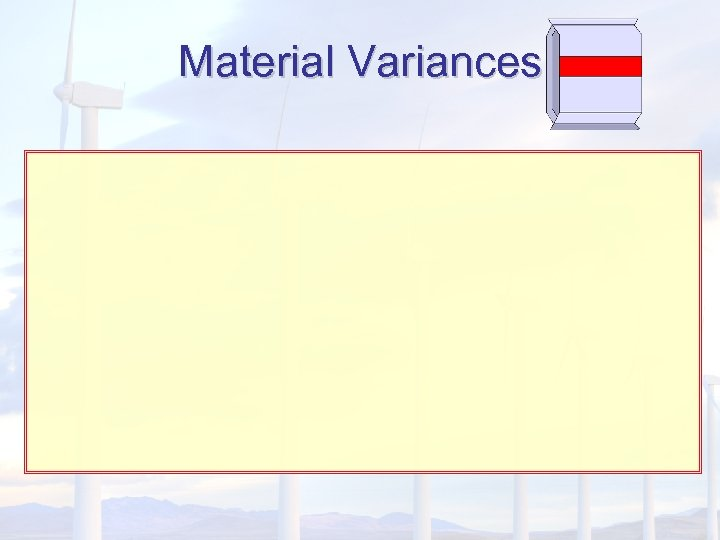Material Variances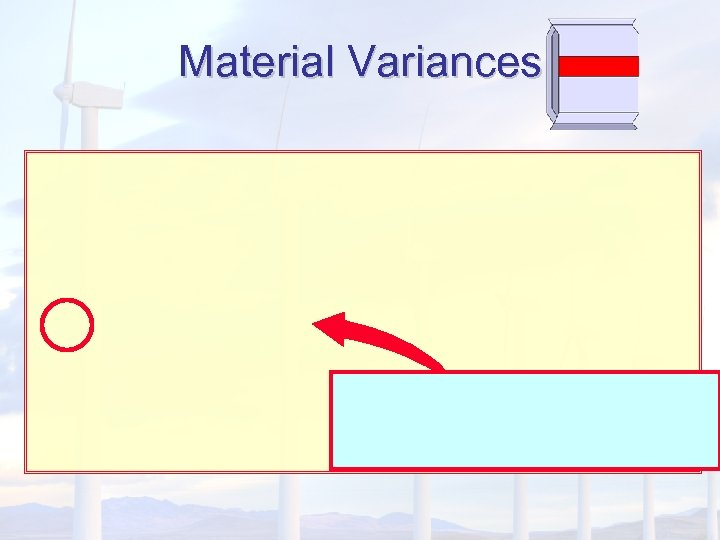Material Variances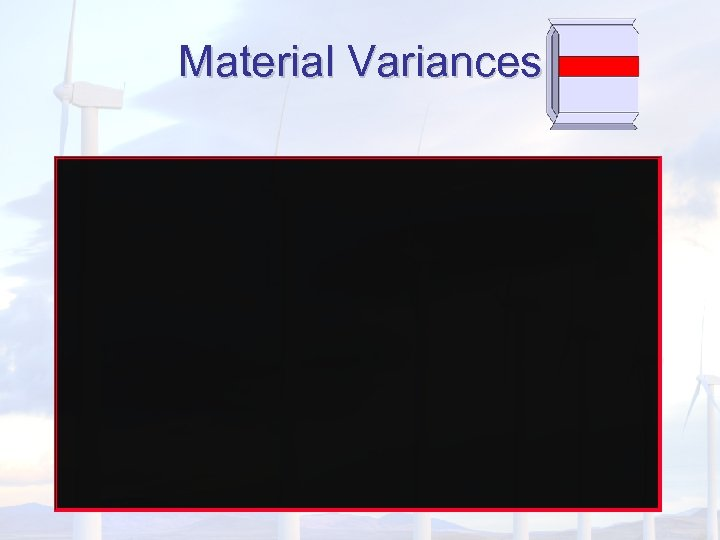Material Variances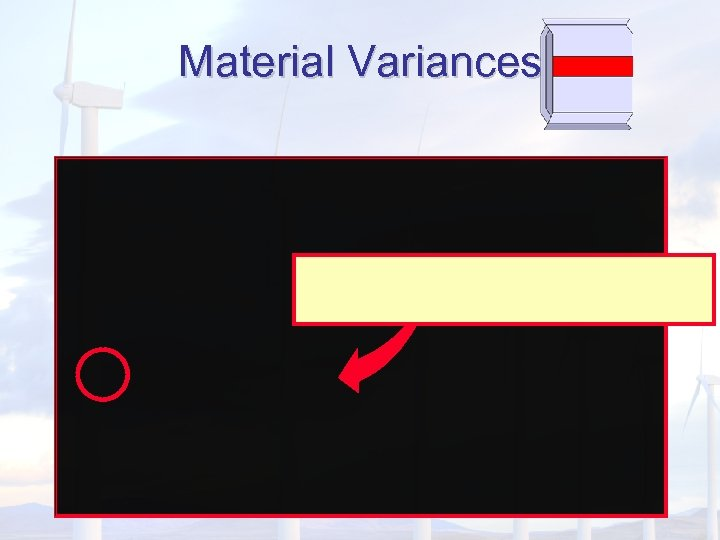Material Variances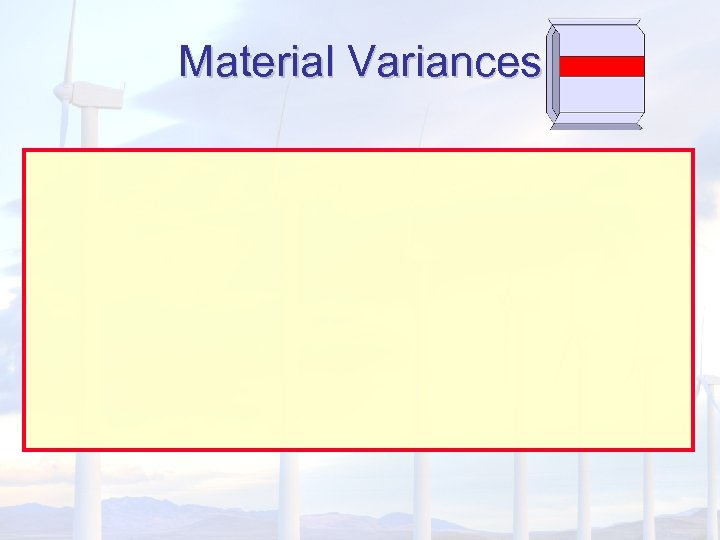Material Variances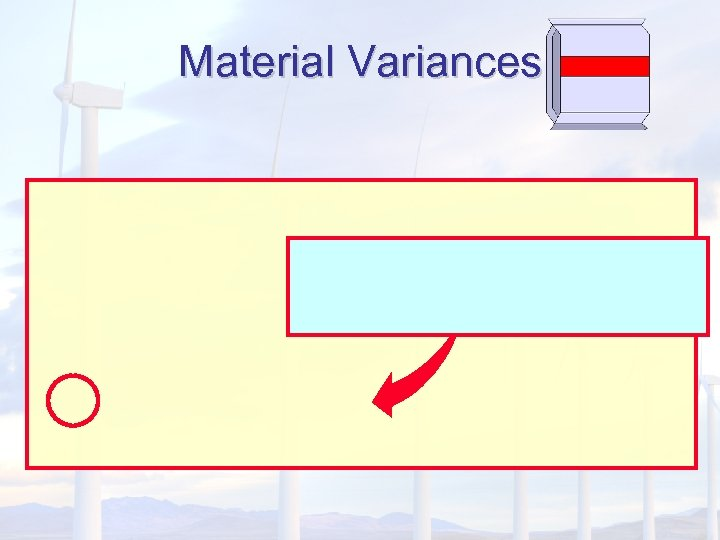Material Variances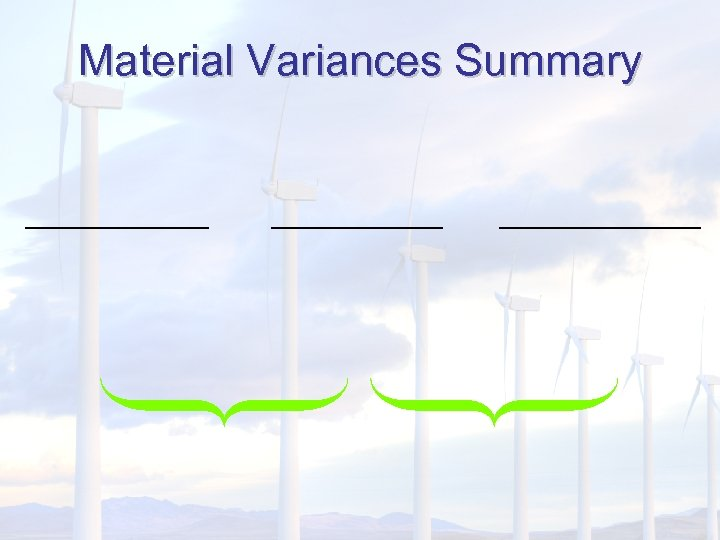Material Variances Summary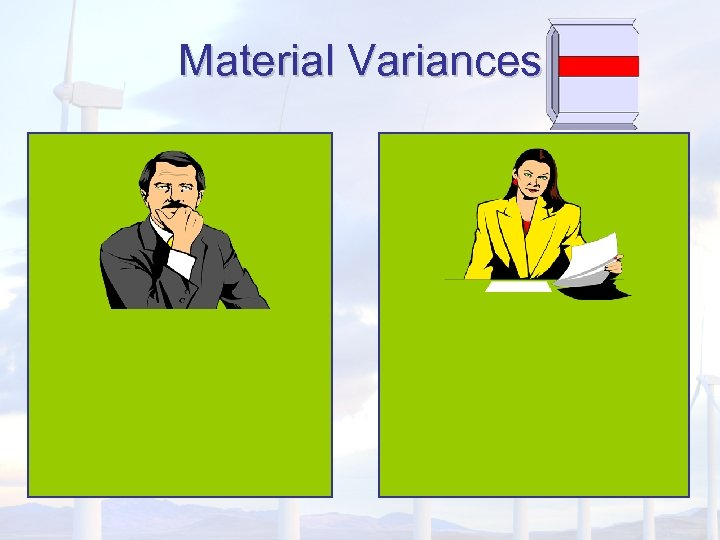Material Variances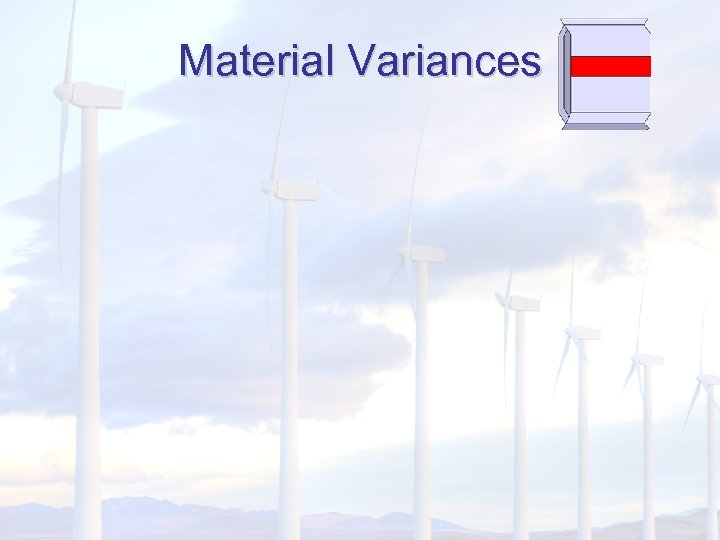Material Variances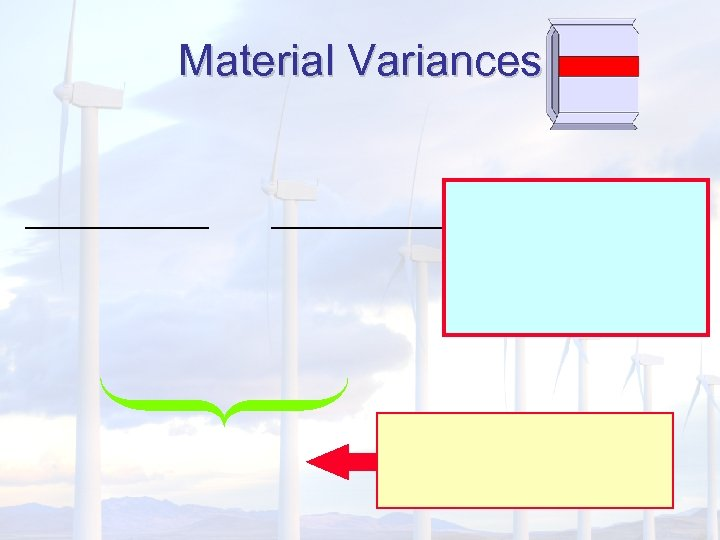Material Variances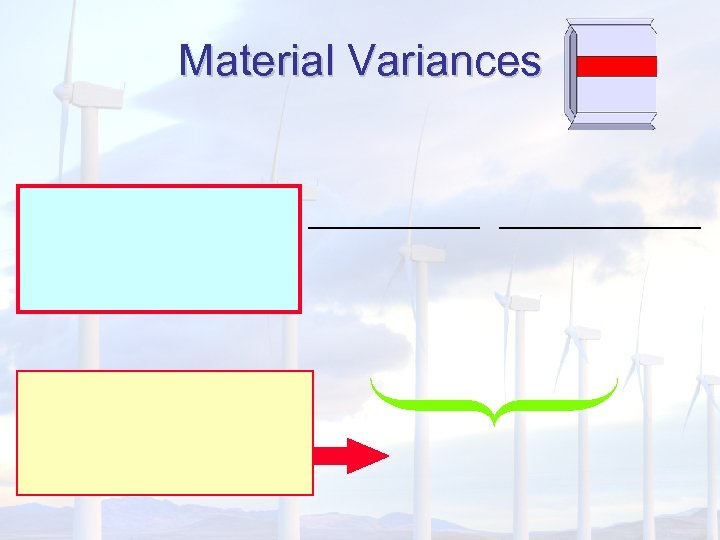Material Variances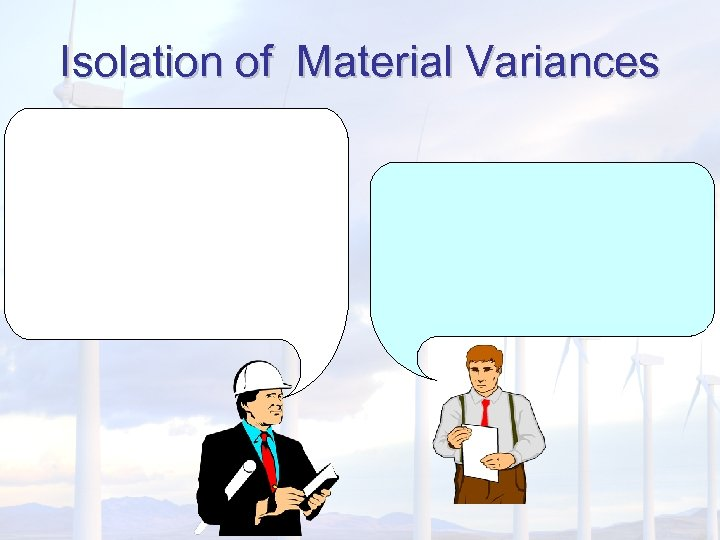Isolation of Material VariancesStandard Costs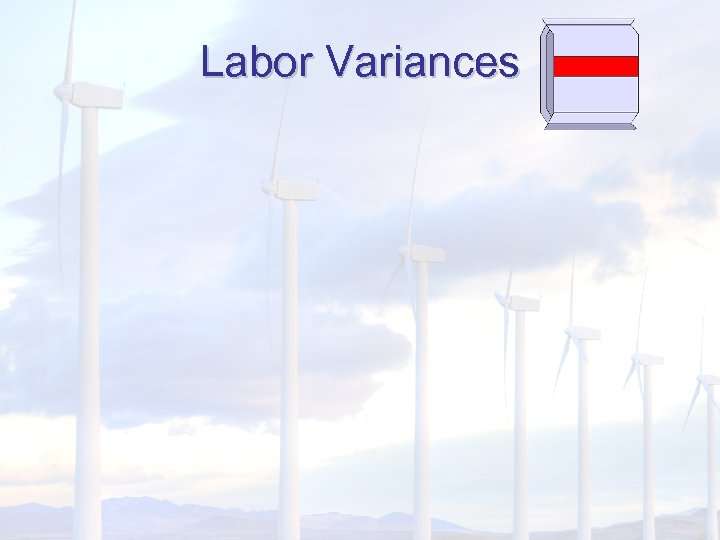Labor Variances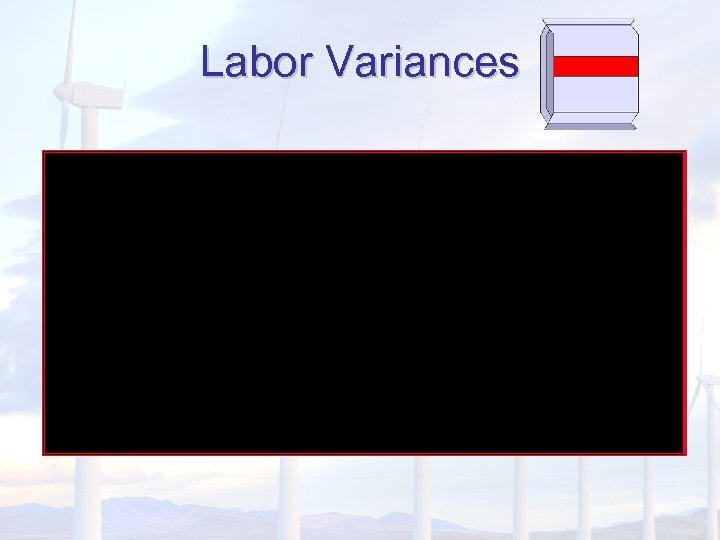Labor Variances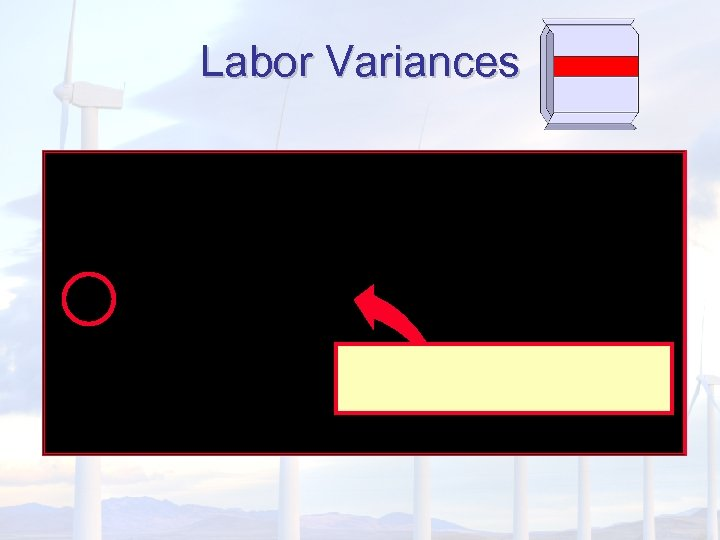Labor Variances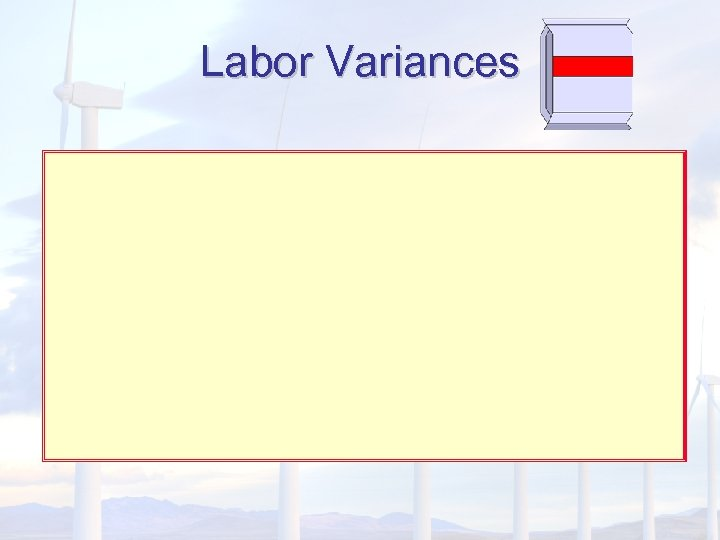Labor Variances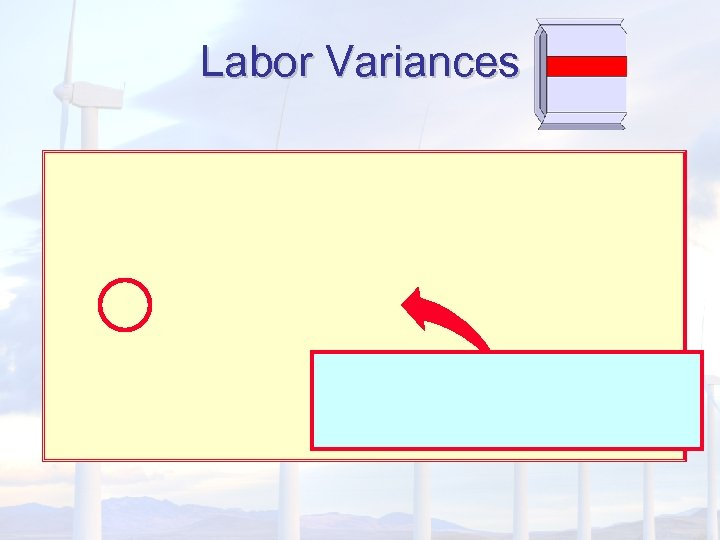Labor Variances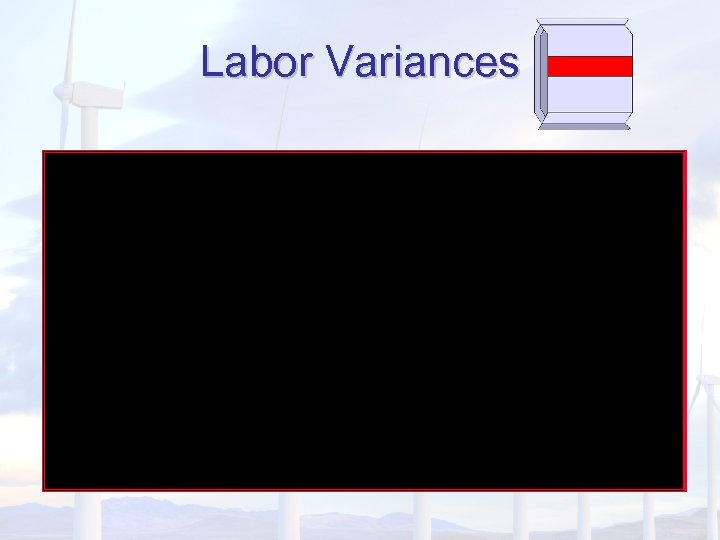Labor Variances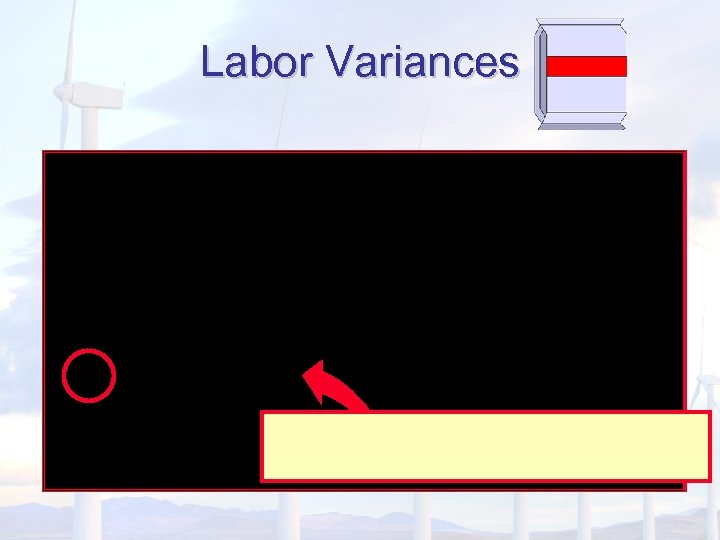Labor Variances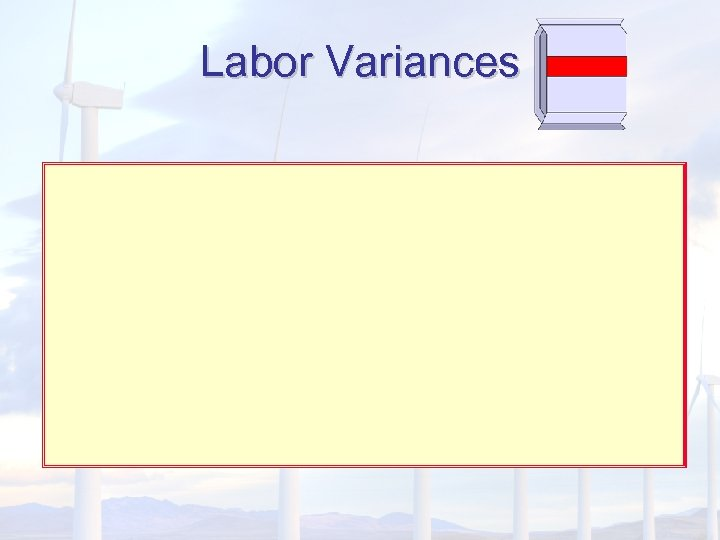Labor Variances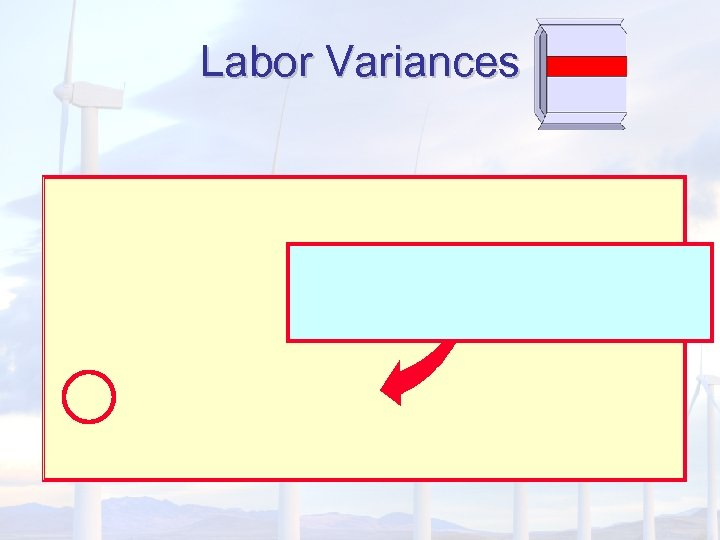Labor Variances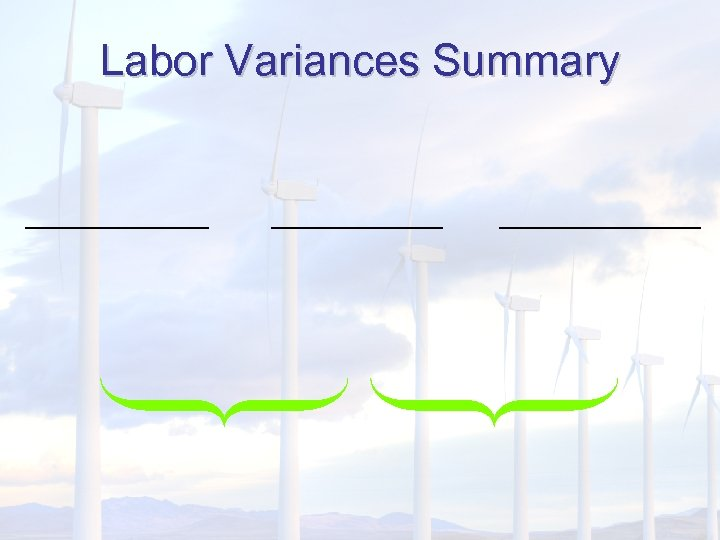Labor Variances Summary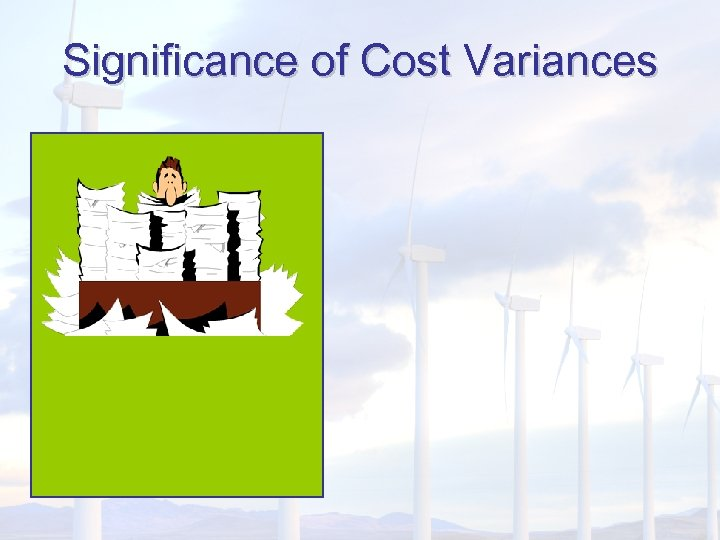Significance of Cost Variances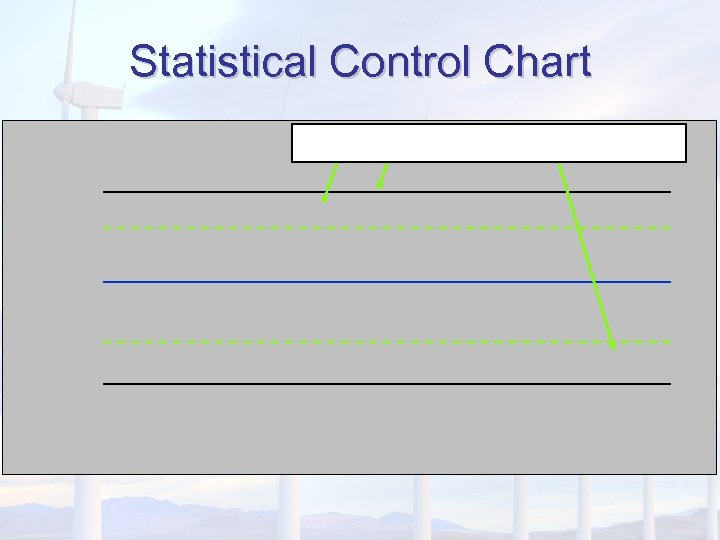Statistical Control ChartBehavioral Impact of Standard Costing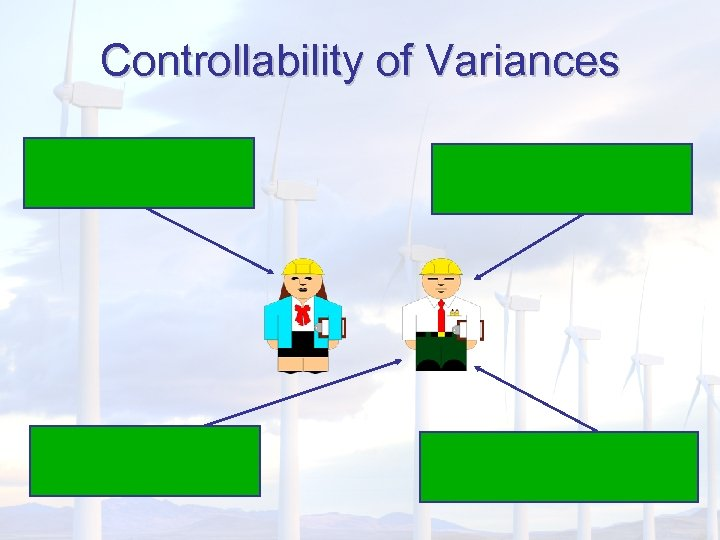Controllability of Variances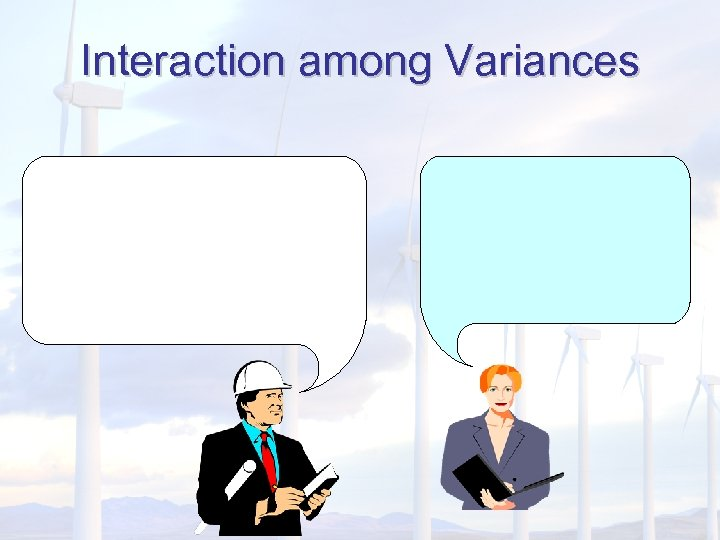Interaction among Variances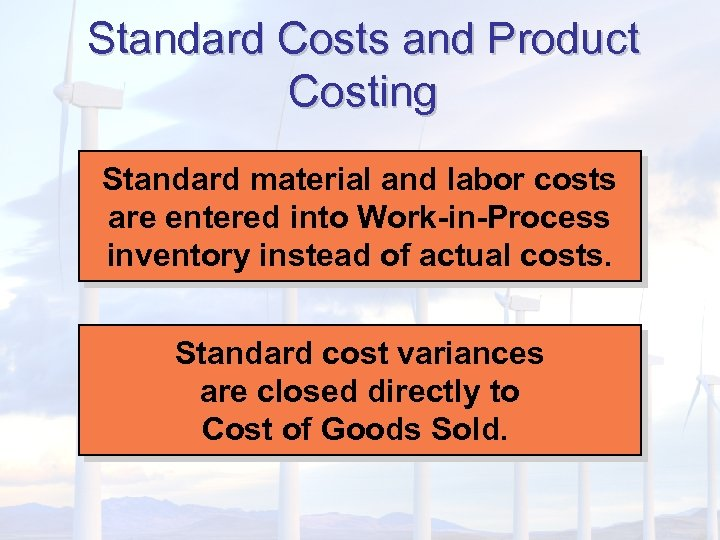Standard Costs and Product Costing Standard material and labor costs are entered into Work-in-Process inventory instead of actual costs. Standard cost variances are closed directly to Cost of Goods Sold.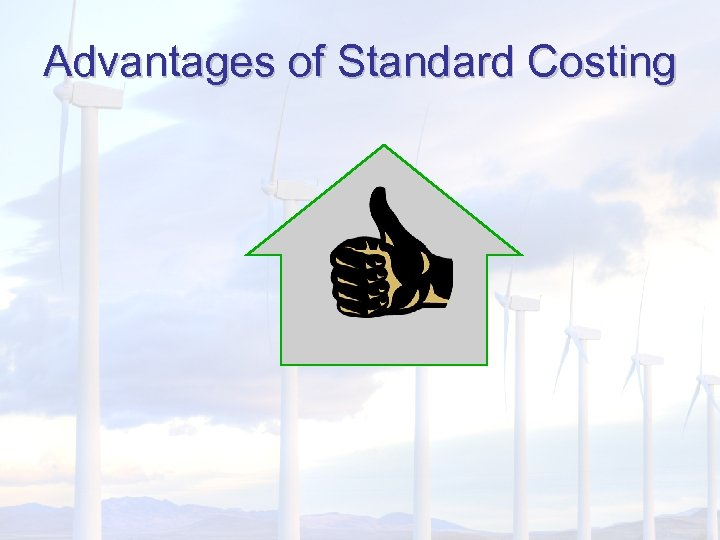Advantages of Standard Costing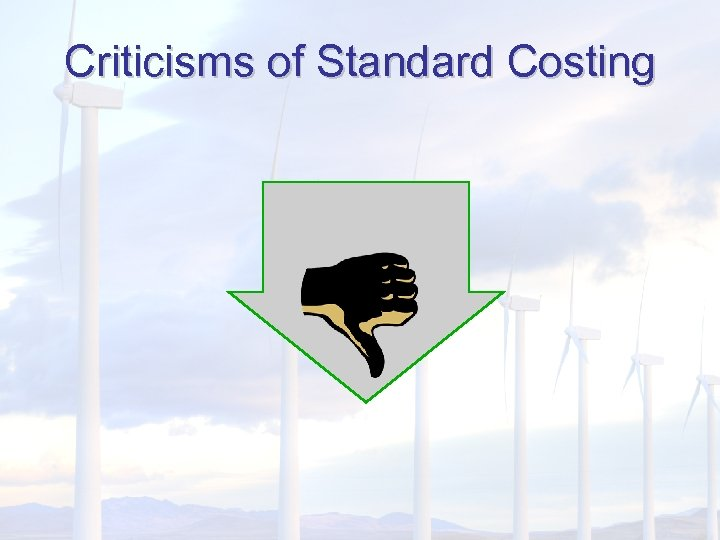Criticisms of Standard Costing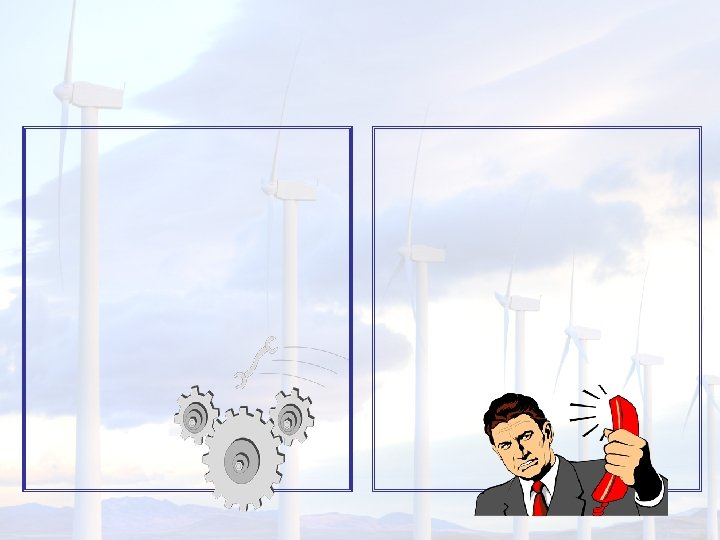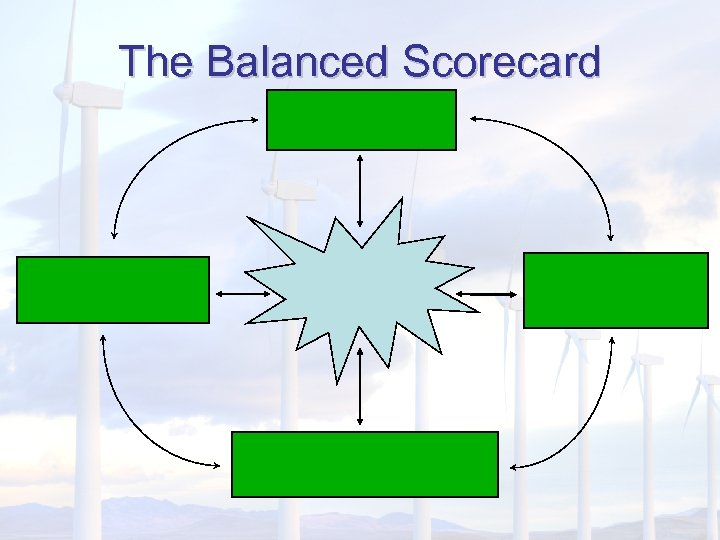The Balanced Scorecard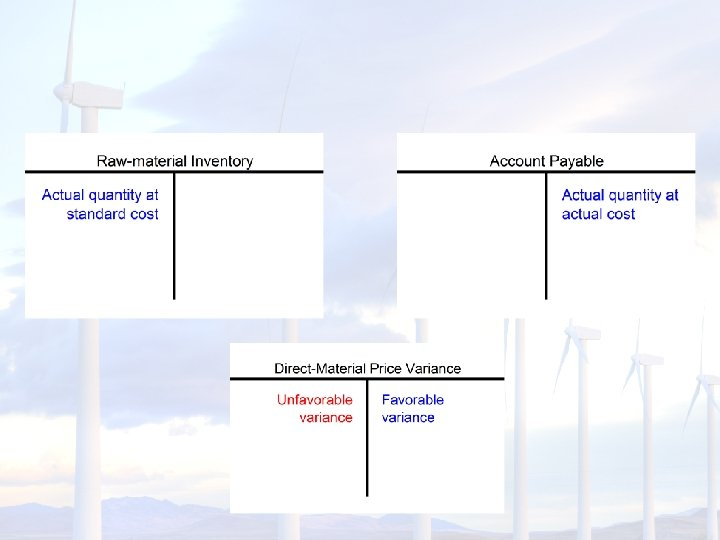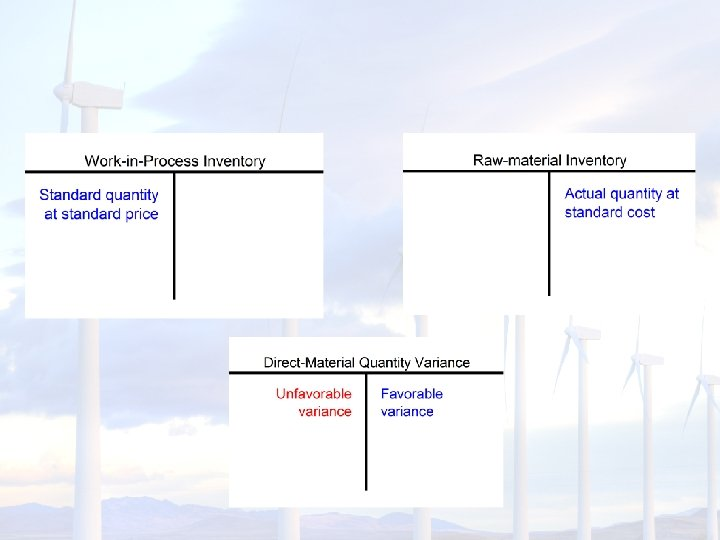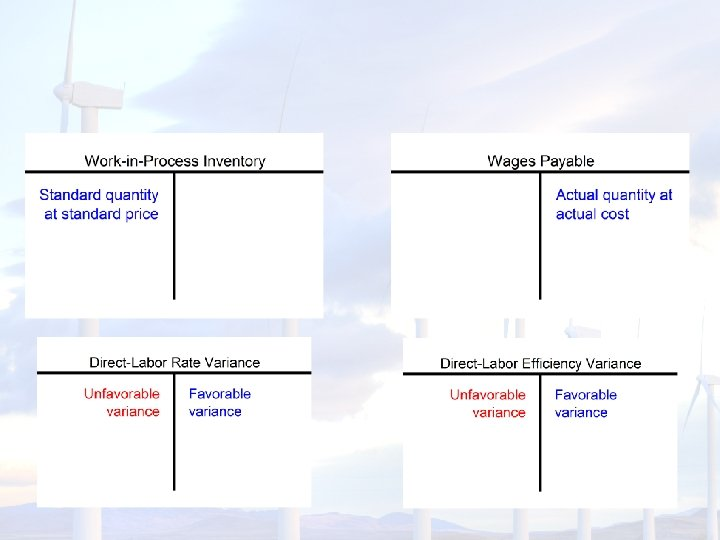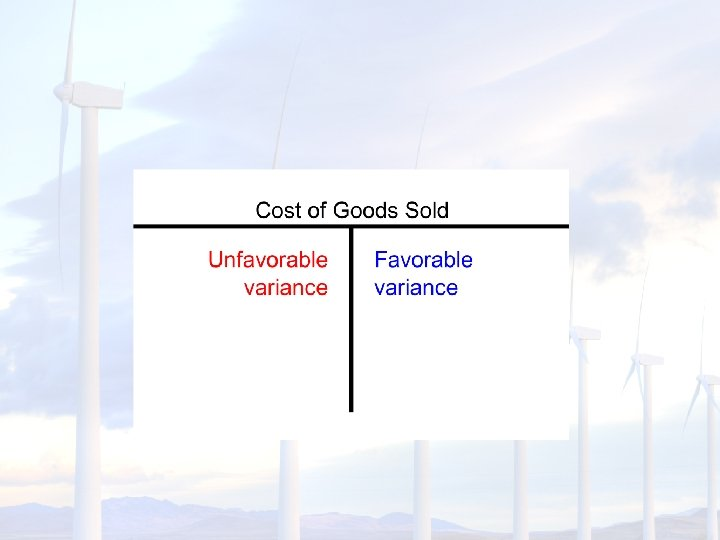End of Chapter 10